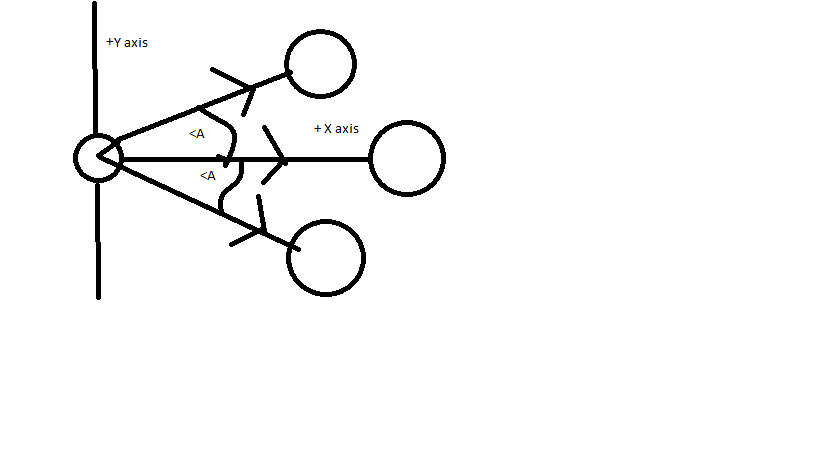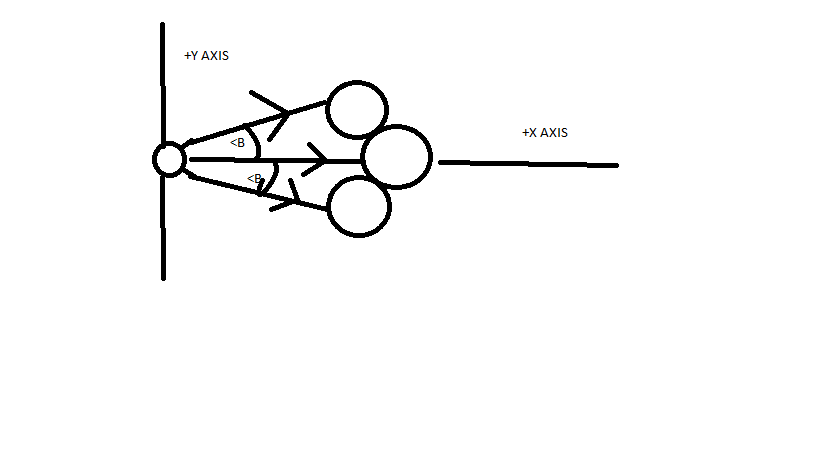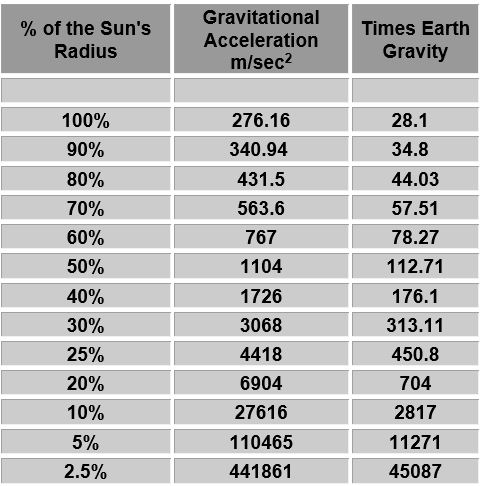### Barisal Zilla School

ABSTRACT:

The paper written by Manzur Rahman of School of Savar which was published in this journal: Correction of Newton of universal gravitation law  was indeed a thought provoking paper.

But there are some points that are invalid in my view. Here I am listing up the problems of his theories.

PROBLEMS

1. The author’s writing is mainly based on a very simple formula of Newton which is mostly part of classical physics. But the experimental results he uses as explanations are somewhat more in depth(containing calculations of Einstein’s relativity and Quantum physics) than this formula and not even his proposed formula can explain these incidents.

Author wrote:

“The picture that we now have from Oppenheimer’s work is as follows. The gravitational field of the star changes the paths of light rays in space-time from what they would have been had the star not been present. The light cones, which indicate the paths followed in space and time by flashes of light emitted from their tips, are bent slightly inward near the surface of the star. This can be seen in the bending of light from distant stars observed during an eclipse of the sun. As the star contracts, the gravitational field at its surface gets stronger and the light cones get bent inward more. This makes it more difficult for light from the star to escape, and the light appear dimmer and redder to an observer at a distance. Eventually, when the star has shrunk to a certain critical radius, the gravitational field at the surface becomes so strong that the light cones are bent inward so much that light can no longer escape which are called Black Holes.”

My point: We know that Photons of light don’t have a mass. So Newtonian formula isn’t applicable to explain this incident. Not even the formula proposed by him (The force of gravitational attraction is directly dependent upon the masses of both objects and there specific gravity and inversely proportional to the square of the distance that separates their centers.) can explain this because of photons having 0 mass.

1. The author’s explanation is mainly based on the internal gravitational force among various particles of a star. Whereas his formula is about gravitational forces between two different bodies(a star and another planet).

Here is a pic I have drawn to show gravity among the particles of a body doesn’t effect the gravitational force among that body and another in the sense the author used it.

PICTURE-1PICTURE-2In Pic-1 the density is low. And we are using a standard model picture for making our calculations easier. In Pic-2 the density is higher. Say the particle in middle lying on x axis is our center of gravity. Now the force applied on the second body by the 1st and 3rd particle of first body from the top, have two components. The y components cancel out each other and the x component remains. Now in Pic-1, the x component of force between either particle-1 or 3 and second body is,( main force in (pic-1) * cos

In second pic, the x component for both are (main force in (pic 2) * cosst  particle is same in mass and even density in both picture 1 and 2 for the sake of argument. So their main force is proportional to the inverse square of distance of particle 1 from the second body. In pic-1 this distance is lower than pic 2. So some trigonometry will show us that.

(Main force in (pic-1)) * cos

This is same for particle 3 in both pic too.

But in the picture-1 internal gravitational force is less than pic-2. But it has no effect at all when we calculate force between two different bodies(Density just determines the center of mass, nothing more).This discussion also clearly shows density has no effect on graviatational force(It just changes the center of gravity but nothing more)

1. The author has given a table but didn’t give clear calculation that he used to determine the values or the source of the table.

Here is the table.I doubt the calculation. Also the distance between center of gravity and earth= Sun’s radius+distance of earth from sun’s surface.. so if we reduce the sun’s radius but keep the distance from sun’s surface same, then value of d^2 decreases and increases the gravity. so there maybe some problems in calculation. If the author shows a proper source for this pic, I will be interested to check that pic up and will try to understand it’s calculation.

1. The author has made a big blunder in the part: “examples”

Here is what he wrote:

“From above calculation, it is clear that sun attract moon more strongly than earth which is slightly above of double (2.2121969).According to newton’s law moon should orbit sun but it don’t. Because of another factor that works behind of these which is specific gravity.”

My view: Actually there are other better explanations that can explain this incident. When three bodies have effect of gravity force on each other, the calculation is much more complex and it requires a clear calculation of relativity which author didn’t produce.

1. Author in the example part did some calculation to find the distance of earth-moon and sun-moon. Now, these formulas usually comes from the newton’s formula itself whereas author is questioning the newton’s formula!! He would have to show the procedure of finding out distance using his hypothesis/formula(The force of gravitational attraction is directly dependent upon the masses of both objects and there specific gravity and inversely proportional to the square of the distance that separates their centers.)

Conclusion: So we decide from the above discussion that author’s discussion is insufficient to prove his hypothesis. He has to clear these 5 points and prove his formula to make it acceptable.